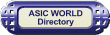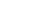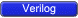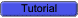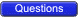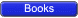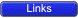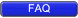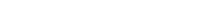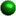Procedural Block Control Procedural blocks become active at simulation time zero. Use level sensitive event controls to control the execution of a procedure.``` 1 module dlatch_using_always(); 2 reg q; 3 4 reg d, enable; 5 6 always @ (d or enable) 7 if (enable) begin 8 q = d; 9 end 10 11 initial begin 12 \$monitor (" ENABLE = %b D = %b Q = %b",enable,d,q); 13 #1 enable = 0; 14 #1 d = 1; 15 #1 enable = 1; 16 #1 d = 0; 17 #1 d = 1; 18 #1 d = 0; 19 #1 enable = 0; 20 #10 \$finish; 21 end 22 23 endmodule ``` You could download file dlatch_using_always.v hereAny change in either d or enable satisfies the event control and allows the execution of the statements in the procedure. The procedure is sensitive to any change in d or enable.Combo Logic using Procedural Coding To model combinational logic, a procedure block must be sensitive to any change on the input. There is one important rule that needs to be followed while modeling combinational logic. If you use conditional checking using "if", then you need to mention the "else" part. Missing the else part results in a latch. If you don't like typing the else part, then you must initialize all the variables of that combo block as soon as it enters.Example - One bit Adder``` 1 module adder_using_always (); 2 reg a, b; 3 reg sum, carry; 4 5 always @ (a or b) 6 begin 7 {carry,sum} = a + b; 8 end 9 10 initial begin 11 \$monitor (" A = %b B = %b CARRY = %b SUM = %b",a,b,carry,sum); 12 #10 a = 0; 13 b = 0; 14 #10 a = 1; 15 #10 b = 1; 16 #10 a = 0; 17 #10 b = 0; 18 #10 \$finish; 19 end 20 21 endmodule ``` You could download file adder_using_always.v hereThe statements within the procedural block work with entire vectors at a time.Example - 4-bit Adder``` 1 module adder_4_bit_using_always (); 2 reg[3:0] a, b; 3 reg [3:0] sum; 4 reg carry; 5 6 always @ (a or b) 7 begin 8 {carry,sum} = a + b; 9 end 10 11 initial begin 12 \$monitor (" A = %b B = %b CARRY = %b SUM = %b",a,b,carry,sum); 13 #10 a = 8; 14 b = 7; 15 #10 a = 10; 16 #10 b = 15; 17 #10 a = 0; 18 #10 b = 0; 19 #10 \$finish; 20 end 21 22 endmodule ``` You could download file adder_4_bit_using_always.v hereExample - Ways to avoid Latches - Cover all conditions``` 1 module avoid_latch_else (); 2 3 reg q; 4 reg enable, d; 5 6 always @ (enable or d) 7 if (enable) begin 8 q = d; 9 end else begin 10 q = 0; 11 end 12 13 initial begin 14 \$monitor (" ENABLE = %b D = %b Q = %b",enable,d,q); 15 #1 enable = 0; 16 #1 d = 0; 17 #1 enable = 1; 18 #1 d = 1; 19 #1 d = 0; 20 #1 d = 1; 21 #1 d = 0; 22 #1 d = 1; 23 #1 enable = 0; 24 #1 \$finish; 25 end 26 27 endmodule ``` You could download file avoid_latch_else.v hereExample - Ways to avoid Latches - Snit the variables to zero``` 1 module avoid_latch_init (); 2 3 reg q; 4 reg enable, d; 5 6 always @ (enable or d) 7 begin 8 q = 0; 9 if (enable) begin 10 q = d; 11 end 12 end 13 14 initial begin 15 \$monitor (" ENABLE = %b D = %b Q = %b",enable,d,q); 16 #1 enable = 0; 17 #1 d = 0; 18 #1 enable = 1; 19 #1 d = 1; 20 #1 d = 0; 21 #1 d = 1; 22 #1 d = 0; 23 #1 d = 1; 24 #1 enable = 0; 25 #1 \$finish; 26 end 27 28 endmodule ``` You could download file avoid_latch_init.v hereSequential Logic using Procedural Coding To model sequential logic, a procedure block must be sensitive to positive edge or negative edge of clock. To model asynchronous reset, procedure block must be sensitive to both clock and reset. All the assignments to sequential logic should be made through nonblocking assignments.Sometimes it's tempting to have multiple edge triggering variables in the sensitive list: this is fine for simulation. But for synthesis this does not make sense, as in real life, flip-flop can have only one clock, one reset and one preset (i.e. posedge clk or posedge reset or posedge preset).One common mistake the new beginner makes is using clock as the enable input to flip-flop. This is fine for simulation, but for synthesis, this is not right.Example - Bad coding - Using two clocks``` 1 module wrong_seq(); 2 3 reg q; 4 reg clk1, clk2, d1, d2; 5 6 always @ (posedge clk1 or posedge clk2) 7 if (clk1) begin 8 q <= d1; 9 end else if (clk2) begin 10 q <= d2; 11 end 12 13 initial begin 14 \$monitor ("CLK1 = %b CLK2 = %b D1 = %b D2 %b Q = %b", 15 clk1, clk2, d1, d2, q); 16 clk1 = 0; 17 clk2 = 0; 18 d1 = 0; 19 d2 = 1; 20 #10 \$finish; 21 end 22 23 always 24 #1 clk1 = ~clk1; 25 26 always 27 #1.9 clk2 = ~clk2; 28 29 endmodule ``` You could download file wrong_seq.v hereExample - D Flip-flop with async reset and async preset``` 1 module dff_async_reset_async_preset(); 2 3 reg clk,reset,preset,d; 4 reg q; 5 6 always @ (posedge clk or posedge reset or posedge preset) 7 if (reset) begin 8 q <= 0; 9 end else if (preset) begin 10 q <= 1; 11 end else begin 12 q <= d; 13 end 14 15 // Testbench code here 16 initial begin 17 \$monitor("CLK = %b RESET = %b PRESET = %b D = %b Q = %b", 18 clk,reset,preset,d,q); 19 clk = 0; 20 #1 reset = 0; 21 preset = 0; 22 d = 0; 23 #1 reset = 1; 24 #2 reset = 0; 25 #2 preset = 1; 26 #2 preset = 0; 27 repeat (4) begin 28 #2 d = ~d; 29 end 30 #2 \$finish; 31 end 32 33 always 34 #1 clk = ~clk; 35 36 endmodule ``` You could download file dff_async_reset_async_preset.v hereExample - D Flip-flop with sync reset and sync preset``` 1 module dff_sync_reset_sync_preset(); 2 3 reg clk,reset,preset,d; 4 reg q; 5 6 always @ (posedge clk) 7 if (reset) begin 8 q <= 0; 9 end else if (preset) begin 10 q <= 1; 11 end else begin 12 q <= d; 13 end 14 15 // Testbench code here 16 initial begin 17 \$monitor("CLK = %b RESET = %b PRESET = %b D = %b Q = %b", 18 clk,reset,preset,d,q); 19 clk = 0; 20 #1 reset = 0; 21 preset = 0; 22 d = 0; 23 #1 reset = 1; 24 #2 reset = 0; 25 #2 preset = 1; 26 #2 preset = 0; 27 repeat (4) begin 28 #2 d = ~d; 29 end 30 #2 \$finish; 31 end 32 33 always 34 #1 clk = ~clk; 35 36 endmodule ``` You could download file dff_sync_reset_sync_preset.v hereA procedure can't trigger itself One cannot trigger the block with a variable that block assigns value or drives.``` 1 module trigger_itself(); 2 3 reg clk; 4 5 always @ (clk) 6 #5 clk = ! clk; 7 8 // Testbench code here 9 initial begin 10 \$monitor("TIME = %d CLK = %b",\$time,clk); 11 clk = 0; 12 #500 \$display("TIME = %d CLK = %b",\$time,clk); 13 \$finish; 14 end 15 16 endmodule ``` You could download file trigger_itself.v hereProcedural Block Concurrency If we have multiple always blocks inside one module, then all the blocks (i.e. all the always blocks and initial blocks) will start executing at time 0 and will continue to execute concurrently. Sometimes this leads to race conditions, if coding is not done properly.``` 1 module multiple_blocks (); 2 reg a,b; 3 reg c,d; 4 reg clk,reset; 5 // Combo Logic 6 always @ ( c) 7 begin 8 a = c; 9 end 10 // Seq Logic 11 always @ (posedge clk) 12 if (reset) begin 13 b <= 0; 14 end else begin 15 b <= a & d; 16 end 17 18 // Testbench code here 19 initial begin 20 \$monitor("TIME = %d CLK = %b C = %b D = %b A = %b B = %b", 21 \$time, clk,c,d,a,b); 22 clk = 0; 23 reset = 0; 24 c = 0; 25 d = 0; 26 #2 reset = 1; 27 #2 reset = 0; 28 #2 c = 1; 29 #2 d = 1; 30 #2 c = 0; 31 #5 \$finish; 32 end 33 // Clock generator 34 always 35 #1 clk = ~clk; 36 37 endmodule ``` You could download file multiple_blocks.v hereRace condition``` 1 module race_condition(); 2 reg b; 3 4 initial begin 5 b = 0; 6 end 7 8 initial begin 9 b = 1; 10 end 11 12 endmodule ``` You could download file race_condition.v hereIn the code above it is difficult to say the value of b, as both blocks are supposed to execute at same time. In Verilog, if care is not taken, a race condition is something that occurs very often.Named BlocksBlocks can be named by adding : block_name after the keyword begin. Named blocks can be disabled using the 'disable' statement.Example - Named Blocks``` 1 // This code find the lowest bit set 2 module named_block_disable(); 3 4 reg [31:0] bit_detect; 5 reg [5:0] bit_position; 6 integer i; 7 8 always @ (bit_detect) 9 begin : BIT_DETECT 10 for (i = 0; i < 32 ; i = i + 1) begin 11 // If bit is set, latch the bit position 12 // Disable the execution of the block 13 if (bit_detect[i] == 1) begin 14 bit_position = i; 15 disable BIT_DETECT; 16 end else begin 17 bit_position = 32; 18 end 19 end 20 end 21 22 // Testbench code here 23 initial begin 24 \$monitor(" INPUT = %b MIN_POSITION = %d", bit_detect, bit_position); 25 #1 bit_detect = 32'h1000_1000; 26 #1 bit_detect = 32'h1100_0000; 27 #1 bit_detect = 32'h1000_1010; 28 #10 \$finish; 29 end 30 31 endmodule ``` You could download file named_block_disable.v hereIn the example above, BIT_DETECT is the named block and it is disabled whenever the bit position is detected.